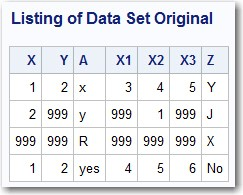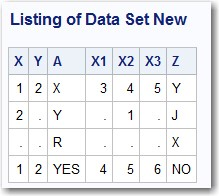In a previous blog, I demonstrated a program and macro that could identify all numeric variables set to a specific value, such as 999. This blog discusses an immensely useful technique that allows you to perform an operation on all numeric or all character variables in a SAS data set. For example, you could convert all numeric variables with a value of 999 to a SAS missing value and all character values to uppercase.

In order to demonstrate how to accomplish these two tasks, first run the program below to create a data set called Original. This data set contains numeric values of 999 and some character values in either lowercase or mixed case:

### Creating a Data Set Called Original

```***Create data set Original; data Original; input X Y A \$ X1-X3 Z \$; datalines; 1 2 x 3 4 5 Y 2 999 y 999 1 999 J 999 999 R 999 999 999 X 1 2 yes 4 5 6 No ;```

Below is a listing of data set Original:

### Listing of Data Set OriginalThe program below searches a SAS data set for all numeric values equal to 999 and converts these values to a SAS missing value.  The program also converts all character values to uppercase.

### Converting all Values of 999 to Missing and converting all Character Values to Uppercase

```data New; set Original; array Nums[*] _numeric_; array Chars[*] _character_; do i = 1 to dim(Nums); if Nums[i] = 999 then Nums[i] = .; end;   do i = 1 to dim(Chars); Chars[i] = upcase(Chars[i]); end; drop i; run;```

You use the two keywords _NUMERIC_ and _CHARACTER_ in the ARRAY statements to start the ball rolling.  When these keywords are used in a DATA step, they refer to all of the numeric or character variables at that point in the DATA step.  This is an important fact.  If you had placed the two ARRAY statements before the SET statement, the two arrays would not contain any variables.

In defining the two arrays, you can use an asterisk (*) instead of the number of elements in the array.  But, because you didn't want to count how many numeric and character variables were in data set Original, you use the DIM (stands for dimension) function that returns the number of elements in an array   You then use this value as the upper limit of your DO loop.

The output is shown below:Notice that the original values of 999 are now missing values and all the character values are in uppercase.  Please think of this technique any time you need to perform an operation on all numeric or character variables in a SAS data set.

SharePrivate Consultant

Ron Cody, EdD is a retired professor from the Robert Wood Johnson Medical School. He now works as a private consultant and a national instructor for SAS Institute Inc. A SAS user since 1977, Ron's extensive knowledge and innovative style have made him a popular presenter at local, regional, and national SAS conferences. He has authored or co-authored numerous books, as well as countless articles in medical and scientific journals.

1.HI,
How can I do proc means in database in only a numeric variable and proc freq in only caractrial variable in big database (3000 variable).

Thank you

2.Thanks Ron. Nice and very useful but you could also, in this context, share the tip on how to use implicit arrays that are easier.
data New;
set Original;
array _Nums _numeric_;
array _Chars _character_;
do over _Nums; if _Nums=999 then _Nums=.; end;
do over _Chars; _Chars = upcase(_Chars); end;
run;